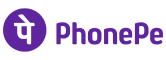New update is available. Click here to update.

# Search In A Sorted 2D Matrix

Medium0/807 upvotes## Problem Statement

#### The following properties apply to the given matrix:

``````1. In each row, integers are sorted from left to right.
2. Each row's first integer is greater than the previous row's last integer.
``````

#### Example:

``````Input:
'MATRIX' = [ [1, 3, 5, 7], [10, 11, 16, 20], [23, 30, 34, 60] ], 'TARGET' = 3
Output:1
Explanation: Since the given number ‘TARGET’ is present in the matrix, we return true.
``````
Detailed explanation ( Input/output format, Notes, Images )
##### Sample Input 1:
``````3 3
1 3 7
10 12 15
19 20 21
12
``````
##### Sample Output 1:
``````1
``````
##### Explanation Of Sample Input 1:
``````Input:
'MATRIX' = [ [1, 3, 7], [10, 12, 15], [19, 20, 21] ], 'TARGET' = 12
Output: 1

Explanation: Since the given number ‘TARGET’ is present in the matrix, we return true.
``````
##### Sample Input 2:
``````4 4
1 5 9 13
14 15 16 17
19 20 21 50
59 60 71 80
80
``````
##### Sample Output 2:
``````1
``````
##### Constraints:
``````1 <= 'N', 'M' <=10^5
1 <= 'MATRIX [ i ] [ j ]', 'TARGET' <= 10^9
The sum of N*M over all test cases is less than 2*10^5.
Time Limit: 1 sec
``````AutocompleteConsole